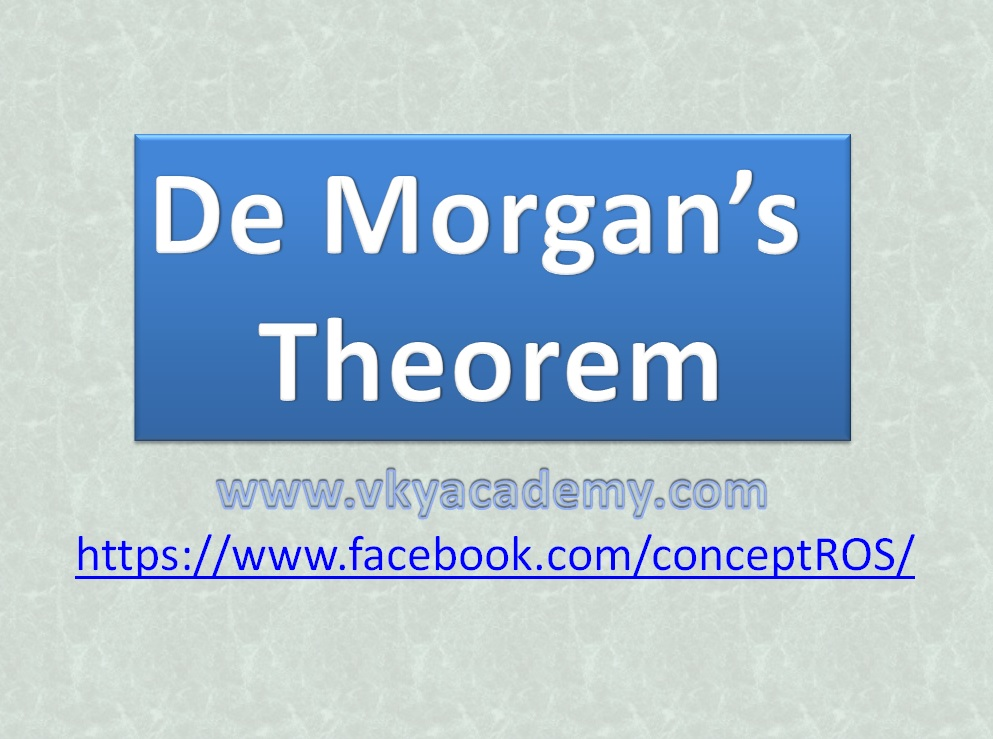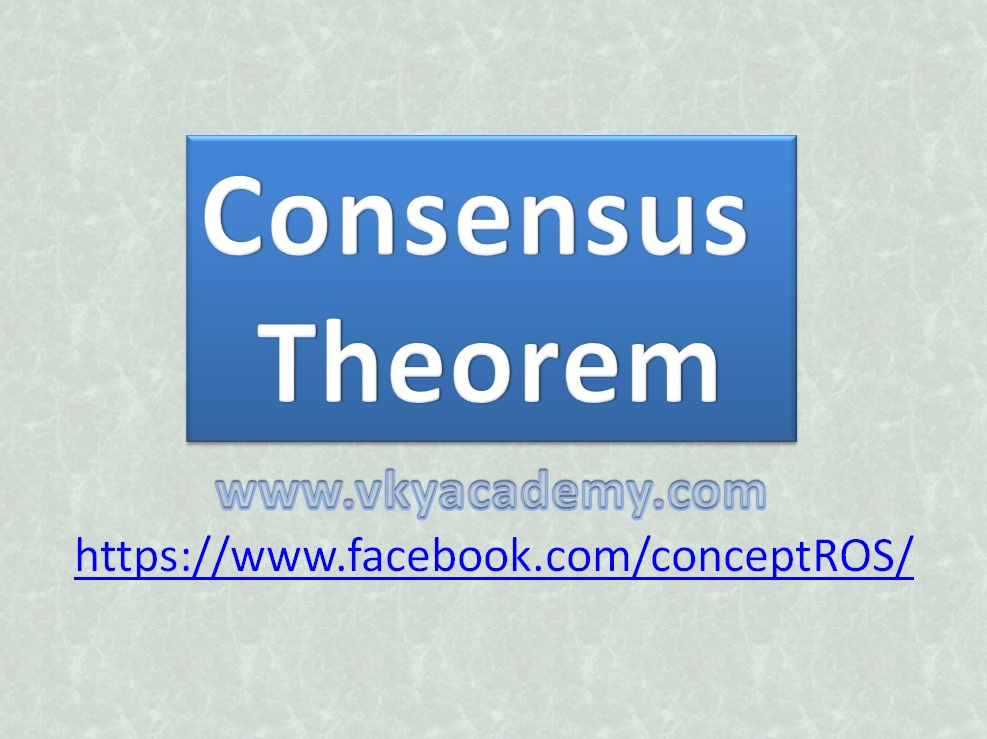# Digital Electronics## De Morgan’s Theorems of Boolean Algebra [Digital Electronics]

In Digital ELectronics there are various laws and rules for the simplification of boolean functions which are basically used to minimize the complexity of digital hardware design by minimizing the boolean function. “De Morgan’s Theorems of Laws” is one of the most powerful theorems used in digital electronics for the minimization of boolean function or …## consensus law Proof/consensus theorem of boolean algebra [Digital Electronics]

In Digital ELectronics there are various laws and rules for the simplification of boolean functions which are basically used to minimize the complexity of digital hardware design by minimizing the boolean function. Consensus Law is one of the most powerful theorems used in digital electronics for the minimization of boolean function or equation either in …## Introduction to Boolean Algebra and Fundamentals of Logic Gates

History and Introduction George Boole, in his work entitled ‘An Investigation of the Laws of Thought’, on which are established the Mathematical Theories of Logic and Probability (1854), presented the fundamental ideas of a two-values (paired) framework called Boolean Algebra. This work was later sorted out and systemized by Claude Shannon in ‘Representative Analysis of …